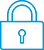cancel
Showing results for
Did you mean:It has been a while since anyone has replied. Simply ask a new question if you would like to start the discussion again.New member
1 0 0 0
Message 1 of 1
540
Flag Post

# Ideas for modern financial calculatorsHP Recommended
HP 12C Platinum Financial Calc

Hello HP community

I am self-taught and self-proclaimed financial engineer having spent the last 10 years research finance formulation. In this regard I have derived many a millions financial formulas that I have published as free to download PDF files from my DropBox account.

Example of my work include:

A generic TVM - time value of money equation with 15 variables which then explodes into 1,700 TVM formulas and since there are 15 variables in the equation thus a total number of roughly 30,000 financial functions

I have thus far written and published 15 books where each such books contains a generic TVM formula followed by list of individual time value of money functions extracted from the said equation

The code is short as one line of programming code is required to write the generic TVM and 1,700 function calls with varying arguments/parameters render the 1,700 values

I wish to ask HP if they would in interested in my current work and if they would be willing to assist me continue my research by a financial backing.

I require small investment money to continue my research

Thanks

Tags (4)
† The opinions expressed above are the personal opinions of the authors, not of HP. By using this site, you accept the Terms of Use and Rules of Participation